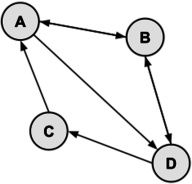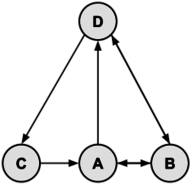## Computer Number System

From helloboyxxx

### 二进制

1 1 0 1## Graph Theory

### Overview

V = {v1,v2,v3,...vn,} 是由 G 的结点 (vertex or node) 组成的集合
E = {e1,e2,e3,...en} 是由连接两个结点的边 (edge) 组成的集合

### 树### 有向图V={A,B,C,D,E}
E={AB,AC,AE,CD,DE,ED,DB}

### 邻接矩阵$\begin{bmatrix} 0 & 1 & 1 & 0 & 1\\ 0& 0 & 0 & 0 & 0\\ 0 & 0 & 0 & 1 & 0\\ 0 & 1 & 0 & 0 & 1\\ 0 & 0 & 0 & 1 & 0 \end{bmatrix}$

### 遍历性A有3条边连接
B有3条边连接
C有5条边连接

## Problems

1. Solve for x where x16=36768.

3. Find the number of different cycles contained in the directed graph with vertices {A, B, C, D, E} and edges {AB, BA, BC, CD, DC, DB, DE}.

4. In the following directed graph, find the total number of different paths from vertex A to vertex C of length 2 or 4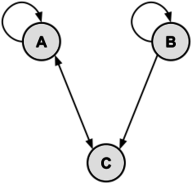5. Given the adjacency matrix, draw the directed graph.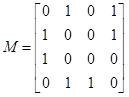## Solutions

1. One method of solution is to convert 36768 into base 10, and then convert that number into base 16 to yield the value of x.
An easier solution, less prone to arithmetic mistakes, is to convert from octal (base 8)to hexadecimal (base 16) through the binary (base 2) representation of the number:

\begin{aligned} 3676_8 &= 011\; 110\; 111\; 110_2\\ &=0111\; 1011\; 1110_2\\ &=7BE_{16} \end{aligned}

1. One could convert the hex numbers into base 10, perform the subtraction, and then convert the answer back to base 16. However, working directly in base 16 isn't too hard. As in conventional decimal arithmetic, one works from right-to-left, from the least significant digits to the most.

The rightmost digit becomes 2, because D-B=2.
The next column is A-E. We need to borrow a one from the 5 column, and 1A-E=C
In the next column, 4-9=B, again, borrowing a 1 from the next column.
Finally, the leftmost column, E-6=8

Combining these results of each column, we get a final answer of 8BC216.

2. The graph is as follows: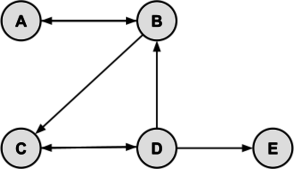By inspection, the cycles are: ABA, BCDB, and CDC. Thus, there are 3 cycles in the graph.

3. Let matrix M represent the graph. Recall that the number of paths from vertex i to vertex j of length p equals Mp. The values of M, M2 and M4 are: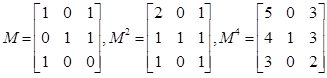There is 1 path of length 2 from A to C (cell [1,3]) and 3 paths of length 4 (cell [1,3]).

4. There must be exactly 4 vertices: V = {A, B, C, D}. There must be be exactly 7 edges: E = {AB, AD, BA, BD, CA, DB, DC}. Here are two valid drawings of the graph: